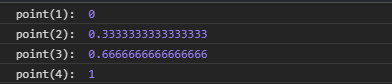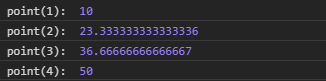Related Articles
D3.js point.domain() Function
• Last Updated : 24 Aug, 2020

The point.domain() function is used to set the domain of the point scale to a specified array of values. The first element in the array is mapped to the first point in the range, the second value in the domain to the second point, and so on.

Syntax:

`point.domain([domain]);`

Parameters: This function accepts single parameters as given above and described below:

• domain: This parameter sets the domain of the point scale i.e the minimum and the maximum value.

Return Values: This function does not return anything.

Below given are a few examples of the function given above.

Example 1:

 `  ` `<``html` `lang` `= ``"en"``>  ` `<``head``>  ` `    ``<``meta` `charset` `= ``"UTF-8"` `/>  ` `    ``<``meta` `name` `= ``"viewport"` `        ``path1tent = "``width``=``device``-width,  ` `        ``initial-scale` `= ``1``.0"/>  ` `    ``<``title``>GeekforGeeks  ` `    ``<``script` `src` `= ` `    ``"https://d3js.org/d3.v4.min.js"``> ` `    `` ` `     `  `  ` `<``style``> ` ` ` `<``body``>  ` `    ``<``script``>  ` `    ``// Creating the point scale with  ` `   ``// specified domain and range. ` `        ``var point = d3.scalePoint() ` `                    ``.domain([1, 2, 3, 4]); ` `       `  `        ``console.log("point(1): ", point(1)); ` `        ``console.log("point(2): ", point(2)); ` `        ``console.log("point(3): ", point(3)); ` `        ``console.log("point(4): ", point(4)); ` `    ``  ` `  ` ``

Output:Example 2:

 `  ` `<``html` `lang` `= ``"en"``>  ` `<``head``>  ` `    ``<``meta` `charset` `= ``"UTF-8"` `/>  ` `    ``<``meta` `name` `= ``"viewport"` `        ``path1tent = "``width``=``device``-width,  ` `        ``initial-scale` `= ``1``.0"/>  ` `    ``<``title``>GeekforGeeks  ` `    ``<``script` `src` `= ` `    ``"https://d3js.org/d3.v4.min.js"``> ` `    `` ` `     `  `  ` `<``style``> ` ` ` `<``body``>  ` `    ``<``script``>  ` `// Creating the point scale with specified domain and range. ` `        ``var point = d3.scalePoint() ` `                    ``.domain([1, 2, 3, 4]) ` `                    ``.range([10, 50]); ` `       `  `        ``console.log("point(1): ", point(1)); ` `        ``console.log("point(2): ", point(2)); ` `        ``console.log("point(3): ", point(3)); ` `        ``console.log("point(4): ", point(4)); ` `    ``  ` `  ` ``

Output:My Personal Notes arrow_drop_up
Recommended Articles
Page :